Case 1: Planetary-rotation Coater

This example shows the well-known planetary-rotation coater, in which substrates are held by fixtures that rotate about their axes, which in turn, orbit around a center axis. The ratio of the two angular speeds is usually a pseudo irrational number for achieving good uniformity.

In this example, the calculated 27 surface points, defined in 'Surface Coated' panel, are along a radius of 25 cm. With the use of two correction masks (coupled) a peak-to-valley nonuniformity of 0.16% is obtained across the radius [please see footnote]. The placement of the masks, at 0 and 120 degrees, allow two material sources at the two different angular locations to produce the same uniform thickness. (The masks are optimized through the mask optimization program in 'Mask Optimizer' panel.) The thicknesses displayed are in unit of 'Nanometers per gram of material evaporated', assuming the material has a density of 3.15 (grams/centimeter cube) for magnesium fluoride.

Also displayed are three histograms of the vapor striking angle – they show the distribution of the incident angles (between 0 to 90 degrees, measured from the surface normal) of the vapor flux at any three surface points of your choosing.

The material utilization is calculated and displayed. In this example, approximately 28% of the evaporated material is collected by the three disks (fixtures) of 25 cm in radius.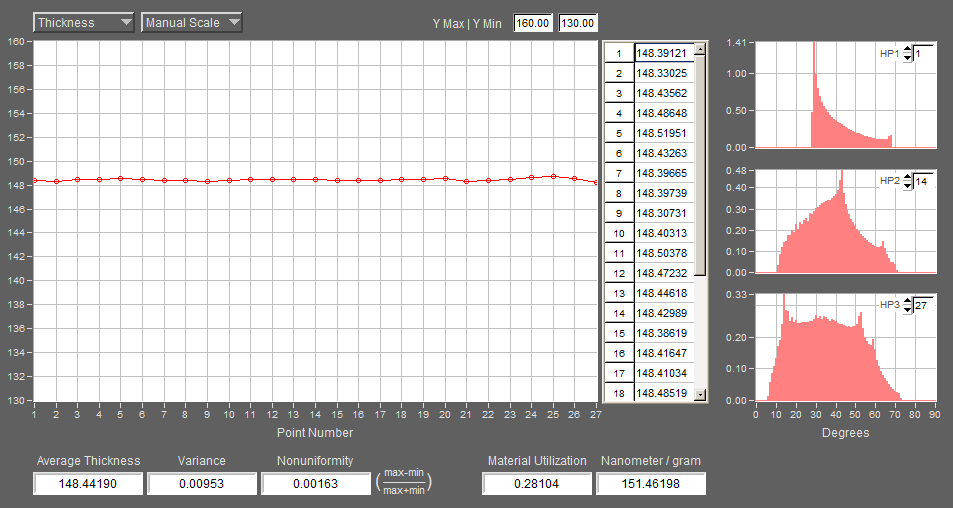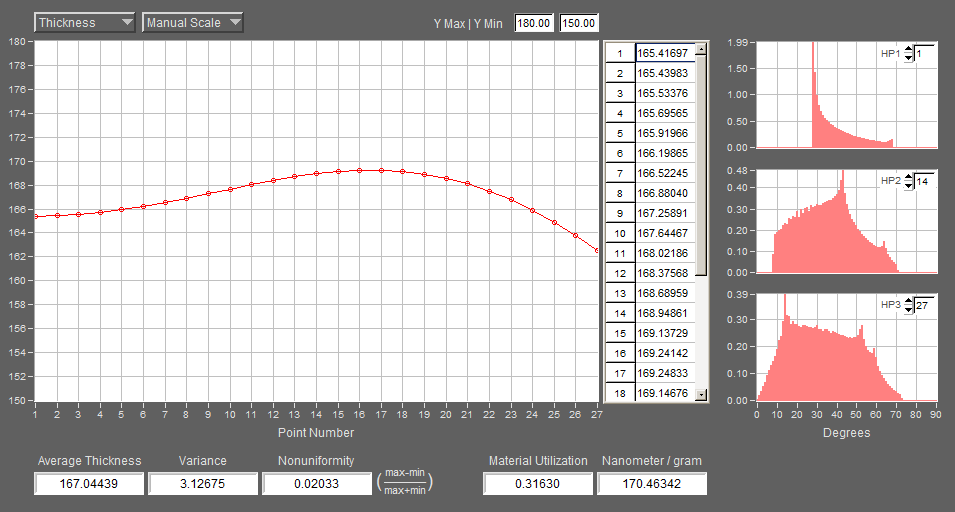Removing the correction masks, the thickness nonuniformity becomes 2.0%; the material utilization becomes 31.6%, as shown below.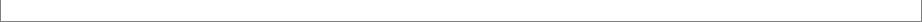Note: In this and other examples we will adopt this definition for thickness nonuniformity:

Nonuniformity = (max-min)/(max+min)

where max and min are the maximum and minimum thickness values in the computed points, respectively. A suitable description for this definition may be "peak-to-valley nonuniformity" with a +/- sign.  For example, if the above formula gives 0.025, we may say the peak-to-valley nonuniformity is +/- 2.5%. For abbreviation, we will omit "+/-" in description.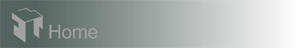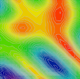Hardware requirements
Request a Free Trial